## Problem #11

For the differential equation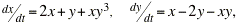(a) Determine all critical points of the given system of equations.

(b) Find the corresponding linear system near each critical point.

(c) Find the eigenvalues of each linear system. What conclusions can you then draw about the nonlinear system?

(d) Draw a phase portrait of the nonlinear system to confirm your conclusions, or to extend them in those cases where the linear system does not provide definite information about the nonlinear system.

## Part (a)

The critical points of this system are (0,0) and (-1.19345, -1.4797), and thus they are both isolated critical points.

## Parts (b) & (c) for (x0, y0) = (0,0)

For functions x' = 2x + y + xy3 = F(x,y) and y' = x - 2y - xy = G(x,y), both F and G are polynomial functions of x and y, so they both have partial derivatives of all orders at any point. Thus, the system is almost linear near each critical point. Near the origin, the differential equation for this problem can be written as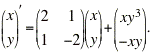The corresponding linear system near the origin is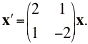The eigenvalues and eigenvectors for this linear system are found using MATLAB's 'eig' command:

```  >> A=[2 1;1 -2];
>> [V,D]=eig(A)
V =
0.2298   -0.9732
-0.9732   -0.2298
D =
-2.2361         0
0    2.2361
>>
```

Thus, the eigenvectors and eigenvalues for the linear system about the origin are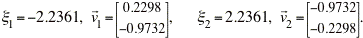The eigenvalues are real, distinct and have opposite signs, so the origin is an unstable saddle point.

## Parts (b) & (c) for (x0, y0) = (-1.19345, -1.4797)

The linear system that approximates the nonlinear system near the critical point (x0, y0) = (-1.9345, -1.4797) is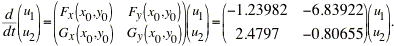We can be glad we have MATLAB to find the eigenvalues and eigenvectors of this system:

```  >> B=[-1.23982 -6.83922;2.4797 -0.80655];
>> [V2,D2]=eig(B)
V2 =
-0.8567            -0.8567
0.0271 + 0.5151i   0.0271 - 0.5151i
D2 =
-1.0232 + 4.1125i        0
0            -1.0232 - 4.1125i
>>
```

Thus, we see that the eigenvalues and eigenvectors are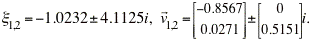Because the eigenvectors are complex with negative real part, the critical point at (-1.19345, -1.4797) is a stable spiral point.

## Part (d)

The module dirfieldAuto.m will plot a direction field for any 2x2 system of autonomous differential equations, and is particularly useful for nonlinear systems such as this one.

```  >> dirfieldAuto
*-----------------------------------------------------------*
| This module will plot a direction field for an autonomous |
| system of equations x' = f(x,y), y' = g(x,y).             |
*-----------------------------------------------------------*

Enter x' as a function of x and y:  x' => 2*x + y + x*y^3
Enter y' as a function of x and y"  y' => x - 2*y - x*y
Enter the smallest x value => -2.5
Enter the largest x value  => 2.5
Enter the smallest y value => -2.5
Enter the largest y value  => 2.5
Warning: Divide by zero.
> In dirfieldAuto at 88
Warning: Divide by zero.
> In dirfieldAuto at 89
>> Would you like to plot a solution curve on this direction field? (y or n) => y
Enter initial condition:
x(0) => 2
y(0) => 1
Would you like to plot another solution curve?  (y or n) => y
```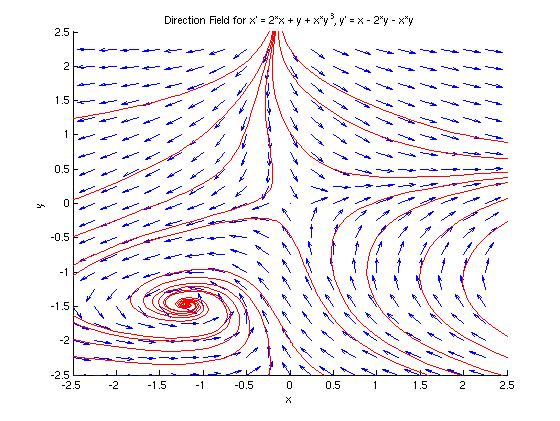We see that the origin is indeed a saddle point, and that there is an inward spiral around the point (-1.19345, -1.4797), as we predicted in the analysis above.